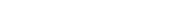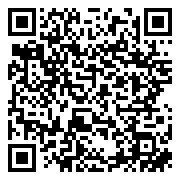# python怎么读取和写入excel表格

【摘要】最近在做一些数据处理和计算的工作，因为数据是以 CSV格式保存的，因此刚开始直接用Excel来处理。但是做着做着发现重复的劳动，其实并没有Python操作Excel，主要用到xlrd和xlwt这两个库，即xlrd是读Excel，xlwt是写Excel的库。

Python写Excel——xlwt（推荐学习：Python视频教程）

Python写Excel的难点，不在构造一个Workbook的本身，而是填充的数据，不过这不在范围内。

``````import xlwt

#设置表格样式
def set_style(name,height,bold=False):
style = xlwt.XFStyle()
font = xlwt.Font()
font.name = name
font.bold = bold
font.color_index = 4
font.height = height
style.font = font
return style

#写Excel
def write_excel():
f = xlwt.Workbook()
row0 = ["姓名","年龄","出生日期","爱好"]
colum0 = ["张三","李四","恋习Python","小明","小红","无名"]
#写第一行
for i in range(0,len(row0)):
sheet1.write(0,i,row0[i],set_style('Times New Roman',220,True))
#写第一列
for i in range(0,len(colum0)):
sheet1.write(i+1,0,colum0[i],set_style('Times New Roman',220,True))

sheet1.write(1,3,'2006/12/12')
sheet1.write_merge(6,6,1,3,'未知')#合并行单元格
sheet1.write_merge(1,2,3,3,'打游戏')#合并列单元格
sheet1.write_merge(4,5,3,3,'打篮球')

f.save('test.xls')

if __name__ == '__main__':
write_excel()```
```

Python读Excel——xlrd

Python读取Excel表格，相比xlwt来说，xlrd提供的接口比较多，但过程也有几个比较麻烦的问题，比如读取日期、读合并单元格内容。

``````import xlrd
from datetime import date,datetime

file = 'test3.xlsx'

wb = xlrd.open_workbook(filename=file)#打开文件
print(wb.sheet_names())#获取所有表格名字

sheet1 = wb.sheet_by_index(0)#通过索引获取表格
sheet2 = wb.sheet_by_name('年级')#通过名字获取表格
print(sheet1,sheet2)
print(sheet1.name,sheet1.nrows,sheet1.ncols)

rows = sheet1.row_values(2)#获取行内容
cols = sheet1.col_values(3)#获取列内容
print(rows)
print(cols)

print(sheet1.cell(1,0).value)#获取表格里的内容，三种方式
print(sheet1.cell_value(1,0))
print(sheet1.row(1).value)```
```

##### 就业培训申请领取|

# 绑定手机号Python编程各地入口iPhone版